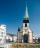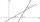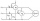# IS triangle

Calculate interior angles of the isosceles triangle with base 38 cm and legs 26 cm long.

Result

α = β =  43 °
γ =  93.9 °

#### Solution:

$\alpha = \beta = \arccos( \dfrac{ 38 }{ 2 \cdot 26} ) =43 ^\circ$
$\gamma = 2 \arcsin( \dfrac{ 38 }{ 2 \cdot 26} ) =93.9 ^\circ$

Leave us a comment of this math problem and its solution (i.e. if it is still somewhat unclear...):Be the first to comment!## Next similar math problems:

1. Right triangleCalculate the missing side b and interior angles, perimeter and area of ​​a right triangle if a=10 cm and hypotenuse c = 16 cm.
2. Trapezoid MOThe rectangular trapezoid ABCD with right angle at point B, |AC| = 12, |CD| = 8, diagonals are perpendicular to each other. Calculate the perimeter and area of ​​the trapezoid.
3. Regular 5-gonCalculate area of the regular pentagon with side 7 cm.
4. Church towerArchdeacon church in Usti nad Labem has diverted tower by 186 cm. The tower is 65 m high. Calculate the angle by which the tower is tilted. Result write in degree's minutes.
5. RectangleCalculate the length of the side GN and diagonal QN of rectangle QGNH when given: |HN| = 25 cm and angle ∠ QGH = 28 degrees.Between cities A and B is route 13 km long of stúpanie average 7‰. Calculate the height difference of cities A and B.
7. Isosceles triangle 10In an isosceles triangle, the equal sides are 2/3 of the length of the base. Determine the measure of the base angles.
8. Two forcesTwo forces with magnitudes of 25 and 30 pounds act on an object at angles of 10° and 100° respectively. Find the direction and magnitude of the resultant force. Round to two decimal places in all intermediate steps and in your final answer.
9. RightDetermine angles of the right triangle with the hypotenuse c and legs a, b, if: ?
10. Ratio iso triangleThe ratio of the sides of an isosceles triangle is 7:6:7 Find the base angle to the nearest answer correct to 3 significant figure.
11. Trigonometric functionsIn right triangle is: ? Determine the value of s and c: ? ?
12. MastMast has 17 m long shadow on a slope rising from the mast foot in the direction of the shadow angle at angle 9.3°. Determine the height of the mast, if the sun above the horizon is at angle 44°30'.
13. SteepleSteeple seen from the road at an angle of 75°. When we zoom out to 25 meters, it is seen at an angle of 20°. What is high?
14. Coordinates of square verticesI have coordinates of square vertices A / -3; 1/and B/1; 4 /. Find coordinates of vertices C and D, C 'and D'. Thanks Peter.
15. Angle between linesCalculate the angle between these two lines: ? ?
16. Sphere in coneA sphere of radius 3 cm desribe cone with minimum volume. Determine cone dimensions.Two wattmeters are connected to measuring power in a 3 phase balanced load. Determine the total power and power factor if the two wattmeters read 1000 watts each (1) both positive and (2) second reading is negative